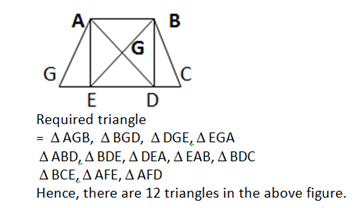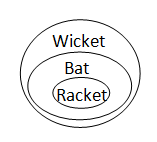# SSC Reasoning Ability Practice Questions – (Day-2)

Dear Aspirants, Here we have given the Important SSC CGL Exams 2018 Practice Test Papers. Candidates, those who are preparing for SSC CGL Exams 2018 can practice these questions to get more confidence to Crack SSC CGL Exams 2018 Examination.

[WpProQuiz 4012]

1) Roushan starts waking from his home facing West direction. After walking 12 km, he takes a right turn and walks another 12 km. He takes another right turn and walks 12 km to reach his school. How far (in km) and in which direction is he from his home?

a) 12 km, North

b) 10 km, South

c) 20 km, North – East

d) 20 km, South – West

2) If 6 × 9 – 4 = 58 and 3 × 9 – 7 = 34, then in the expression A × 4 + 9 = 91, What is the value of A?

a) 6.5

b) 17. 5

c) 20. 5

d) 30. 5

Direction: (3 – 4): In the following questions, select the related word/letter/number from the given alternatives.

3) Car : Steering Wheel : : Motorcycle: ?

a) Tyre

c) Engine

d) Handle bar

4) 0.02 : 0.002 : : 1/3 : ?

a) 0. 333

b) 1/ 30

c) 0.003

d) 1/3

Direction (5 – 6): In the following questions, select the odd word/ letter/ number pair from the given alternatives.

5) BT, DR, FP, ?

a) HO

b) HN

c) NH

d) OH

6) 3, 8, 5, 27, 8, 64, 12, 125, 17, ?

a) 216

b) 361

c) 625

d) 441

7) In a certain code language “who are you” is written as “432”, “they is you” is written as “485” and “they are dangerous” is written as “295”. How is “dangerous” written in that code language?

a) 2

b) 4

c) 5

d) 9

8) Arrange the given words in the sequence in which they occur in the dictionary

1. Strom
2. Strap
3. Strangle
4. Stamped
5. Satire

a) 51432

b) 51342

c) 54132

d) 53412

9) A piece of paper is folded and punched as shown below in the question figures. From the given answer figures, indicate how it will appear when opened?

Question Figures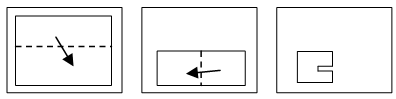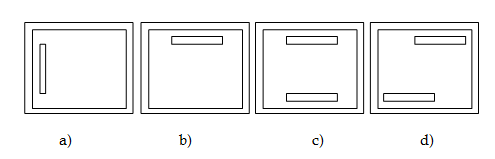10) A word is represented by only one set of numbers as given in anyone of the alternatives. The sets of numbers given in the alternatives are represented by two classes of alphabets as shown in the given two matrices. The columns and rows of Matrix-I are numbered from 0 to 4 and that of Matrix-II are numbered from 5 to 9. A letter from these matrices can be represented first by its row and next by its column, for example, ‘S’ can be represented by 21, 43 etc. and ‘0’ can be represented by 65, 88 etc. Similarly, you have to identify the set for the word ‘SPEAK’.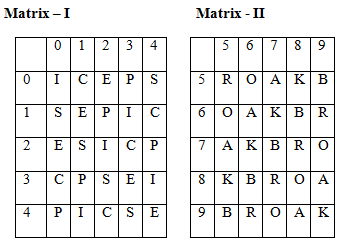a) 10, 12, 11, 66, 58t

b) 43, 31, 33, 89, 86

c) 21, 40, 44, 56, 99

d) 32, 03, 20, 97, 66

11) The ratio of the ages of man and his wife is 4 : 3. After 4 yr, the ratio will be 9 : 7. If at the time of marriage, the ratio was 5 : 3, how many years ago were they married?

a) 12

b) 5

c) 24

d) 8

12) If it is Saturday on 27th September, what day will it be on 27th October of the same year?

a) Thursday

b) Sunday

c) Friday

d) Wednesday

13) In the following question, which one set of letters when sequentially placed at the gaps in the given letter series shall complete it?

a_bc_a_bcdabc_da_cd_

a) acbddb

c) cabddc

d) ddcbbc

14) How many triangles are there in the given figure?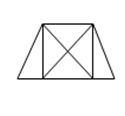a) 8

b) 10

c) 12

d) 14

15) In the following question below are given some statements followed by some conclusions. Taking the given statements to be true even if they seem to be at variance from commonly known facts, read all the conclusions and then decide which of the given conclusion logically follows the given statements?

Statements:

1. All rackets are bats.
2. All bats are wickets.

Conclusion:

1. Some wickets are rackets
2. All wickets are rackets.

a) Only I follow

b) Only II follow

c) Both Follow

d) Neither I nor II follow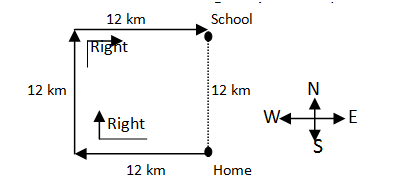As, (6 × 9) + 4 = 54 + 4 = 58

And (3 × 9 ) + 7 = 27 + 7 = 34

A × 4 + 9 = 91

4A = 91 –  9 = 100

A = 82 / 4 = 20.5

Direction (3-4) :

Steering wheel is related to car. Similarly, handle bar is related to motorcycle.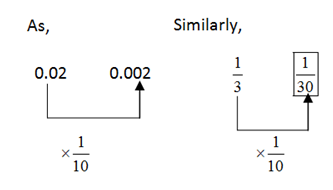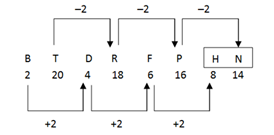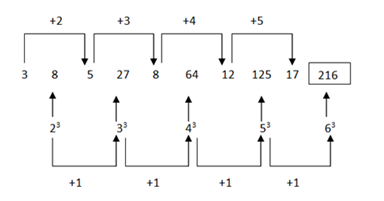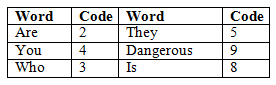Dictionary order is: Satire > Stamped > Storm > Strangle > Storm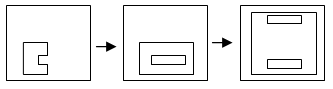SPEAK = > 10, 12, 11, 66, 58

Let present age of man = 4x yr

Present age of his wife             = 3x yr

After 4 years = (4x + 4) / (3x + 4) = 9 / 7

= 28x + 28 = 27x + 36

28x – 27x = 36 – 28

X = 8

Present age of Man = 4x = 4 * 8 = 32

Present age of his wife = 3x = 3* 8 = 24

Let they got married before y year

(32 – y) / (24 – y) = 5 /3

96 – 3y = 120 – 5y

5y – 3y = 120 – 96

2y = 24 => y = 12

27th September = > Saturday

30th September => Tuesday

1st October => Wednesday

Other Wednesday in October = 8, 15, 22 and 29

October 27 = > Wednesday -2 = Monday

aabcd/abbcd/ abccd/ abcdd